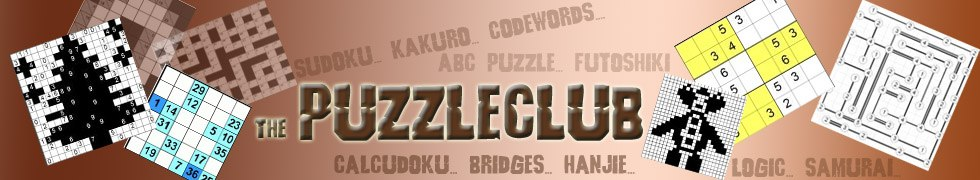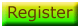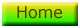SkyScraper Puzzles

Skyscraper puzzles require you to place the numbers from 1 - 6 in each of the rows and columns of the puzzles just once (the numbers vary depending on the dimensions of the puzzle).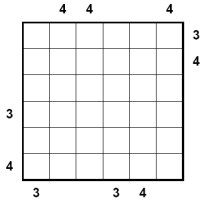The start of the puzzle position looks similar to that on the right, possibly with some numbers given at the start of the puzzle. What you will notice immediately is numbers around the edge of the puzzle. These numbers are the key to solving skyscraper puzzles, and are what give the puzzle its interesting sounding name.

The numbers around the outside tell you how many buildings you would be able to see in that row or column from that vantage point. Thus in a 6x6 puzzle, if there is a 1 at the start of a row, then you know that the 6 must be placed first in that row, because 6 is the 'tallest' building (the highest number). If, however, there was a 2 at the start of a row, then you know that the 6 cannot be the first in the row because otherwise it would block out all other buildings. So, you need to convert numbers to the size of skyscrapers, so a building with six floors would tower above a building with just one floor for instance. In other words, the numerical value of the numbers is essential to solving this puzzle in a way that is not the case in sudoku where the numbers can be replaced with symbols of fruits or colours and so on without affecting the logic of the puzzle.

We have a new selection of 50 skyscraper puzzles on this page for members to play. If you are logged in, then the links to all of these puzzles will appear beneath this introduction to the skyscraper puzzles. They are in PDF format for you to print and play when you like complete with solutions. If you're not a member, then you can Register here to access these skyscraper puzzles and thousands of other puzzles here at the Puzzle Club.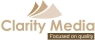©   |     |